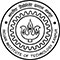How to use a CASE statement in SQL

# How to use a CASE statement in SQL

clickHere
Updated on Nov 22, 2022 19:35 IST

In SQL you can apply conditions on the cell based on other cells using CASE statement. In this article we will discuss how to use a case statement in sql in different scenarios.

## What is a SQL CASE statement?

SQL CASE statement is similar to the IF-THEN-ELSE statement of any other programming language that returns the result after evaluating conditions. If none of the conditions is evaluated to TRUE, it will return the condition given in the ELSE block.

Syntax

` `
`CASEWHEN condition_1 THEN value_1WHEN condition_2 THEN value_2……WHEN condition_n THEN value_nELSE valueENDCopy code`

Points to Remember:

• CASE statement must be followed by at least one WHEN-THEN statement
• ELSE argument is optional
• NULL value can’t be checked using CASE statement

Now, let’s move to some examples to get a better understanding of how to use a CASE statement in SQL.

Example -1: Below table (Student) contains the marks of ten students, using a case statement give each student a grade.

Now, we will define grade for each students using CASE statement.

Query

` `
`SELECT *    CASE          WHEN Percentage > 90 THEN ‘O’          WHEN Percentage > 80 AND Percentage <= 90 THEN ‘A’          WHEN Percentage > 70 AND Percentage <= 80 THEN ‘B’          WHEN Percentage > 60 AND Percentage <= 70 THEN ‘C’          WHEN Percentage > 50 AND Percentage <= 60 THEN ‘D’         ELSE ‘Fail’      END AS Grade,FROM Student;Copy code`

Output

Must Read: Difference between SQL and NoSQL

### CASE with GROUP BY

Example 2: Using the above Student data, classify the students in to Pass or Fail and count the number of student in both the category.

Query

` `
`SELECT    CASE       WHEN Percentage > 50 THEN ‘PASS’      ELSE ‘FAIL’    END AS Category,    COUNT (*) AS Total _number_of_studentsFROM StudentGROUP BY Grade;Copy code`

Output

### ORDER BY with CASE

Example 3: Sort the result of example 1 in Descending order i.e. sort the student marks such that highest grades are on top?

Query

` `
`SELECT Name    CASE          WHEN Percentage > 90 THEN ‘O’          WHEN Percentage > 80 AND Percentage <= 90 THEN ‘A’          WHEN Percentage > 70 AND Percentage <= 80 THEN ‘B’          WHEN Percentage > 60 AND Percentage <= 70 THEN ‘C’          WHEN Percentage > 50 AND Percentage <= 60 THEN ‘D’         ELSE ‘Fail’      END AS Grade,FROM StudentORDER BY Percentage DESC;Copy code`

Output

Now, in the next example we will use GROUP BY, ORDER BY and COUNT together

Example 4: Find the number of student in each grade.

Query

` `
`SELECT    CASE          WHEN Percentage > 90 THEN ‘O’          WHEN Percentage > 80 AND Percentage <= 90 THEN ‘A’          WHEN Percentage > 70 AND Percentage <= 80 THEN ‘B’          WHEN Percentage > 60 AND Percentage <= 70 THEN ‘C’          WHEN Percentage > 50 AND Percentage <= 60 THEN ‘D’         ELSE ‘Fail’      END AS Grade,      COUNT(*) AS Total StudentFROM StudentGROUP BY GradeORDER BY Percentage DESC;Copy code`

Output

Must Check: SQL Online Course and Certifications

## Conclusion

In this article, we have discussed how to use a CASE statement in SQL with the help of examples.

clickHere

Vikram has a Postgraduate degree in Applied Mathematics, with a keen interest in Data Science and Machine Learning. He has experience of 2+ years in content creation in Mathematics, Statistics, Data Science, and Mac... Read Full Bio

## Trending Management CoursesData Analytics
Great Learning4.2Coursera4.7Excel Basics for Data Analysis
Coursera4.7## Top Picks & New Arrivals